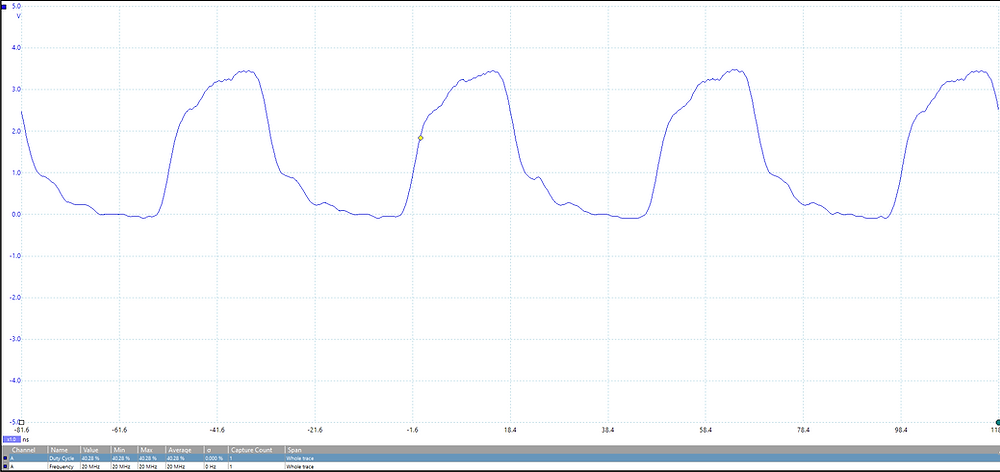Search

# MicroZed Chronicles: A Blast from the Past!

Modern FPGA devices really spoil us. They include PLLs, DCM, DSP etc. and a range of interfaces that significantly ease our developments. Recently though, I faced a situation where the PLL could not be used for safety reasons. This got me scratching my head a little about how I was going to generate a 20 MHz clock from a 50 MHz source without a PLL and also without changing the oscillator frequencies since the 50 MHz is supplied by another satellite payload in this case.Looking back in the notes I’ve gathered over the years, I remembered that the great Peter Alfke published several circuits that could be used in this situation to provide non-integer divisions and clock multiplication. These techniques often come in handy so I thought it would be good to refresh a couple of these techniques and show the performance in modern silicon (Basys 3 Artix-7 FPGA board).

Let’s start with looking at a simple clock doubler circuit. This circuit uses an XNOR gate to generate pulses for each edge of the input clock. As a delay is required to do this in one of the inputs of the XNOR gate, this is provided by the delay register. This logic design will of course not output a 50:50 duty cycle waveform.

By implementing this in a Basys 3 board and providing the clock to the circuit at 10 MHz, we would expect a 20 MHz output routed to the pins of the Pmod output pin. Careful selection of the drive strength, slew rate, pull down, and termination settings presented a reasonable waveform.In addition to the clock multiplication circuit, Peter also presented several division circuits, a divide by 1.5, 2.5, 3 and 5. All of these are based on the use of synchronous counters and then a latch circuit to generate the output.

All of these can be implemented easily in VHDL or Verilog. For the following examples, I used a 10 MHz clock to demonstrate the division algorithms. The first implementation is a divide by 1.5, followed by a divide by 2.5, 3 and 5. The division by 3 and 5 also provide a 50:50 duty cycle.

We should expect to see frequencies like the following when working with a 10 MHz input clock:The RTL for the multiplier and counter as implemented is shown below.

library IEEE;

use IEEE.STD_LOGIC_1164.ALL;

use IEEE.NUMERIC_STD.ALL;

entity top is port(

clk : in std_logic;

clk_1d5 : out std_logic;

clk_2d5 : out std_logic;

clk_3 : out std_logic;

clk_5 : out std_logic;

clk_op : out std_logic

);

end top;

architecture Behavioral of top is

signal inv : std_logic := '0';

signal delay : std_logic := '0';

signal clk_double : std_logic :='0';

signal clk_2d5_cnt : unsigned(2 downto 0) := (others => '0');

signal clk_1d5_cnt : unsigned(1 downto 0) := (others => '0');

signal clk_3_cnt : unsigned(1 downto 0) := (others => '0');

signal clk_5_cnt : unsigned(2 downto 0) := (others => '0');

begin

clk_delay : process(clk_double)

begin

if rising_edge(clk_double) then

delay <= inv;

end if;

end process;

inv <= not delay;

clk_double <= clk XNOR inv;

clk_op <= clk_double;

delay_1_5 : process(clk)

begin

if rising_edge(clk) then

if clk_1d5_cnt = 2 then

clk_1d5_cnt <= (others => '0');

else

clk_1d5_cnt <= clk_1d5_cnt + 1;

end if;

end if;

end process;

clk_1d5 <= '1' when clk_1d5_cnt = 0 and clk ='1' else

'1' when clk_1d5_cnt = 1 and clk ='1' else

'1' when clk_1d5_cnt = 1 and clk ='0' else

'1' when clk_1d5_cnt = 2 and clk ='0' else

'0';

delay_2_5 : process(clk)

begin

if rising_edge(clk) then

if clk_2d5_cnt = 4 then

clk_2d5_cnt <= (others => '0');

else

clk_2d5_cnt <= clk_2d5_cnt + 1;

end if;

end if;

end process;

clk_2d5 <= '1' when clk_2d5_cnt = 1 and clk ='1' else

'1' when clk_2d5_cnt = 1 and clk ='0' else

'1' when clk_2d5_cnt = 3 and clk ='0' else

'1' when clk_2d5_cnt = 4 and clk ='1' else

'0';

delay_3 : process(clk)

begin

if rising_edge(clk) then

if clk_3_cnt = 2 then

clk_3_cnt <= (others => '0');

else